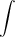# Evaluate Line Integral using Calculus Made Easy

How to Evaluate the following line integral, where C is the given curve.C

y3ds, C: x = t3, y = t, 0 ≤ t ≤ 3

please follow this straightforward approach:
1) Select Vector Calculus under F6
2) F2 2 Line Integral and enter

f=y^3 as integrand
variables [x,y]
Now lets enter the path next:
[t^3,t]
variable : t
bounds : [0,3]

Press ENTER a few times and VOILA !!
Easy as pie.